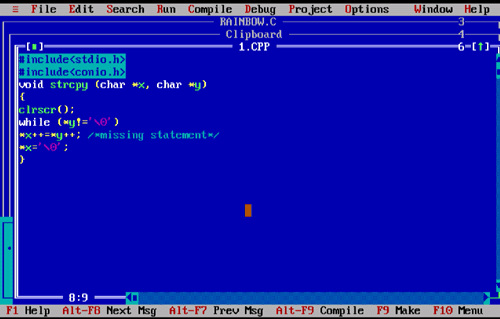# What is the missing statement in the following function which copies string x into string y?

```	void strcpy (char *x, char *y)
{
while (*y!='\0')
................./*missing statement*/
*x='\0';
}
```

What will be the result of execution?

A x=y
B *x++=*y++
C (*x)++=(*y)++
D none of these
Option: [B]

Pointer variable char *x is pointing to a location and the char *y is assigned to that location. If we assume the missing statement is *x++=*y++ then both the variables point to the next respective location till null ('\0') found.